Courses

# Design Of Shoe Brakes Mechanical Engineering Notes | EduRev

## Mechanical Engineering : Design Of Shoe Brakes Mechanical Engineering Notes | EduRev

The document Design Of Shoe Brakes Mechanical Engineering Notes | EduRev is a part of the Mechanical Engineering Course Machine Design.
All you need of Mechanical Engineering at this link: Mechanical Engineering

Types of brakes

Brakes are devices that dissipate kinetic energy of the moving parts of a machine. In mechanical brakes the dissipation is achieved through sliding friction between a stationary object and a rotating part. Depending upon the direction of application of braking force, the mechanical brakes are primarily of three types

• Shoe or block brakes – braking force applied radially
• Band brakes – braking force applied tangentially.
• Disc brake – braking force applied axially.

2. Shoe or block brake

In a shoe brake the rotating drum is brought in contact with the shoe by suitable force. The contacting surface of the shoe is coated with friction material. Different types of shoe brakes are used, viz., single shoe brake, double shoe brake, internal expanding brake, external expanding brake. These are sketched in figure 12.1.1.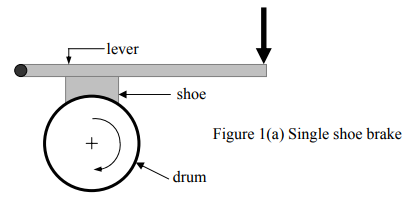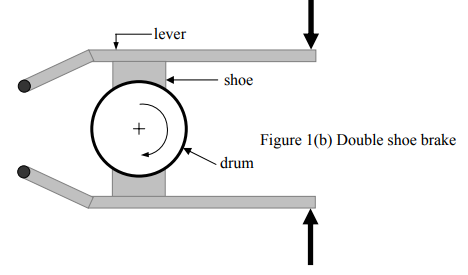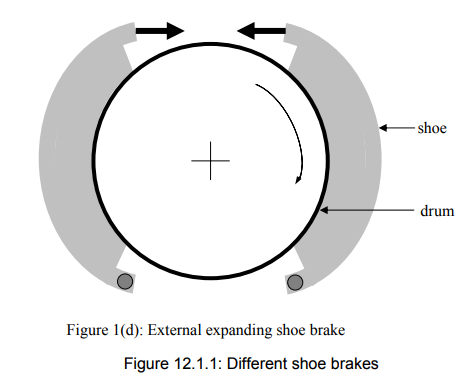Single Shoe brake The force needed to secure contact is supplied by a lever. When a force F is applied to the shoe (see figure 12.1.2a ) frictional force proportional to the applied force ' Ffr = μ' F develops, where μ ' depends of friction material and the geometry of the shoe. A simplified analysis is done as discussed below.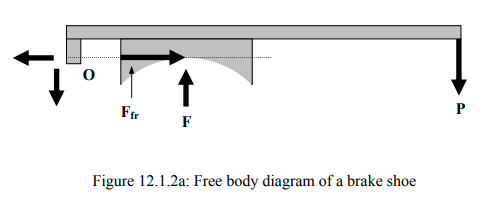Though the exact nature of the contact pressure distribution is unknown, an approximation (based on wear considerations) is made as

p (θ) = pcos θ

Where the angle is measured from the centerline of the shoe. If Coulomb’s law of friction is assumed to hold good, then

ffr (θ) = μp0 cos θ

Since the net normal force of the drum is F, one has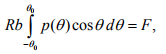Where R and b are the radius of the brake drum and width of the shoe respectively.
The total frictional torque is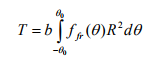If the total frictional force is assumed to be a concentrated one, then the equivalent force becomes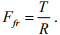A simple calculation yields,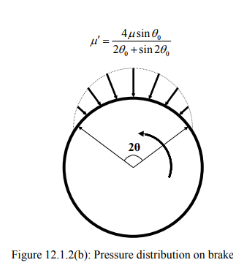It may be seen that for very small value of  θ0 ,μ = μ '. Even when ,  θ0 = 300 μ ' =  1.0453 .  Usually if the contact angle is below , the two values of friction coefficient are taken to be equal. 600

Consider, now single shoe brakes as shown in figures 12.1.3(a) and 3(b). Suppose a force P is applied at the end of a lever arm with length l. The shoe placed at a distance x from the hinge experiences a normal force N and a friction force F, whose direction depends upon the sense of rotation of the drum. Drawing free body diagram of the lever and taking moment about the hinge one gets

(a) for clockwise rotation of the brake wheel,

Nx + Fa = Pl

(b) for anticlockwise rotation of the brake wheel,

Nx – Fa = Pl.

Where a is the distance between the hinge and the line of action of F and is measured positive when F acts below point O as shown in the figure. Using Coulomb’s law of friction the following results are obtained,

(a) for clockwise rotation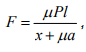(b) for anticlockwise rotation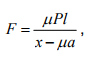It may be noted that for anticlockwise rotating brake, if μ > x/a, then the force P has negative value implying that a force is to applied in the opposite direction to bring the lever to equilibrium. Without any force the shoe will, in this case, draw the lever closer to the drum by itself. This kind of brake is known as ‘selflocking, brake. Two points deserve attention.

(1) If a < 0, the drum brake with clockwise rotation becomes self-energizing and if friction is large, may be self locking.

(2) If the brake is self locking for one direction, it is never self locking for the opposite direction. This makes the self locking brakes useful for ‘back stop’s of the rotors.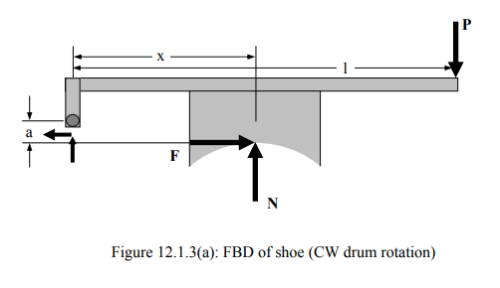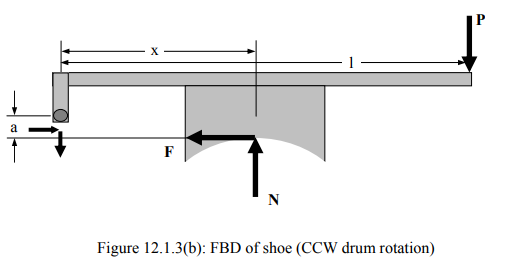Double shoe brake

Since in a single shoe brake normal force introduces transverse loading on the shaft on which the brake drum is mounted two shoes are often used to provide braking torque. The opposite forces on two shoes minimize the transverse loading. The analysis of the double shoe brake is very similar to the single shoe brake.

External expanding shoe brake

An external expanding shoe brake consists of two symmetrically placed shoes having inner surfaces coated with frictional lining. Each shoe can rotate about respective fulcrum (say, O1 and  O2). A schematic diagram with only one shoe is presented (figure 12.1.4) When the shoes are engaged, non-uniform pressure develops between the friction lining and the drum. The pressure is assumed to be proportional to wear which is in turn proportional to the perpendicular distance from pivoting point (O1N in figure 12.1.4). A simple geometrical consideration reveals that this distance is proportional to sine of the angle between the line joining the pivot and the center of the drum and the line joining the center and the chosen point. This means

p (θ) =  psin θ,

where the angle is measured from line OOand is limited as θ1 ≤ θ ≤ θ2 . Drawing the free body diagram of one of the shoes (left shoe, for example) and writing the moment equilibrium equation about (say) the following equation is resulted for clockwise rotation of the drum :

F1l  = M2 - Mf ,

Where is the force applied at the end of the shoe, and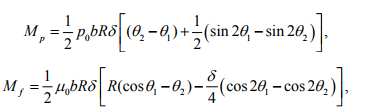where δ is the distance between the center and the pivot (OO1 in figure 12.1.4) and A is the distance from the pivot to the line of action of the force F1 (O1C in the figure). In a similar manner the force to be applied at the other shoe can be obtained from the equation

F2l  = Mp - Mf

The net braking torque in this case is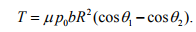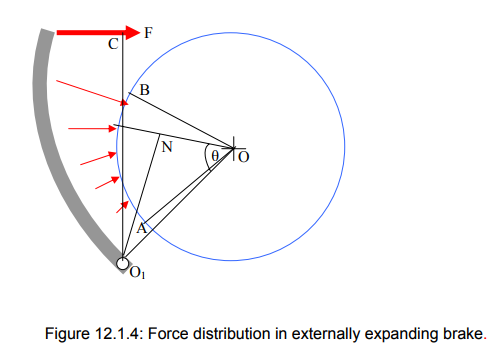Internal expanding shoe brake

Here the brake shoes are engaged with the internal surface of the drum. The analysis runs in the similar fashion as that of an external shoe brake. The forces required are

F1= (Mp+Mf) / l

and F= (Mp+Mf) / l

respectively. One of the important member of the expanding shoe brakes is the anchor pin. The size of the pin is to be properly selected depending upon the face acting on it during brake engagement.

Offer running on EduRev: Apply code STAYHOME200 to get INR 200 off on our premium plan EduRev Infinity!

## Machine Design

57 videos|71 docs|102 tests

,

,

,

,

,

,

,

,

,

,

,

,

,

,

,

,

,

,

,

,

,

;